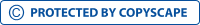Consumer theory, Demand, ElasticityView larger

# Consumer theory, Demand, Elasticity

546740

• College
• 276

Short excerpt:

1.The price of elasticity of demand for paint is simply the percentage change in quantity demanded over the percentage change in price. In this case, the quantity demanded drops from 35 gallons a month to 20 gallons. For this portion of the equation we have the new demand (20 gallons) and take away the old demand (35 gallons). Then, divide the result by the old demand (35 gallons). For example:The result of this equation is -0.42857. Now that we have this number, we must discover the percentage change in price. The old price was \$3.00 while the new price is \$3.50. First of all, we must get the new price (3.50) and take away the old price (3.00). Once we have done this, we can divide by the old price (3.00). For example:The result of this equation is 0.1666. We take the percentage changeBy buying this product you can collect up to 11 loyalty points. Your cart will total 11 loyalty points that can be converted into a voucher of \$0.11.

\$1.10